# 5. A work station is shown below. The station is operated 16 hours a day for...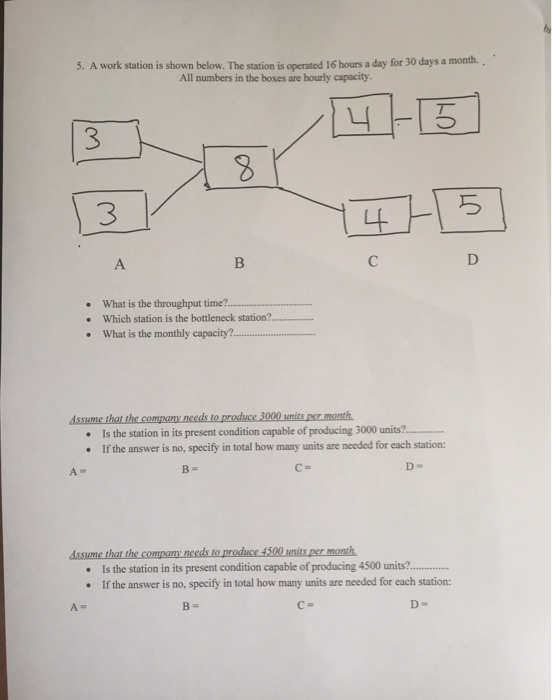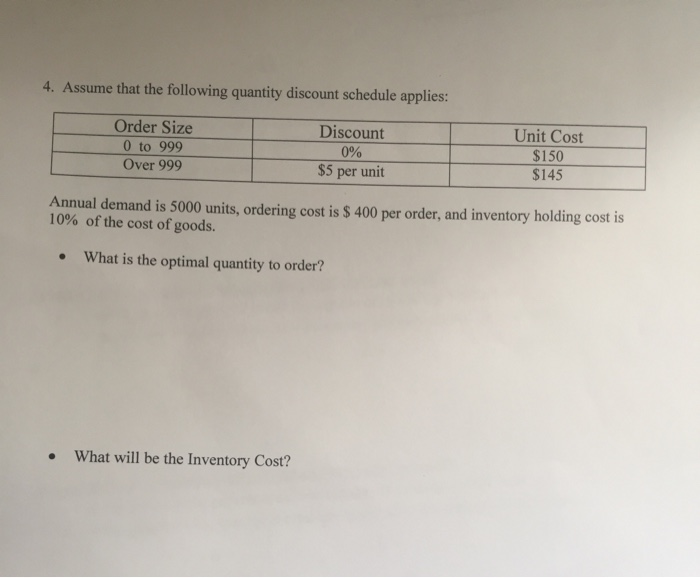5. A work station is shown below. The station is operated 16 hours a day for 30 days a month.. All numbers in the boxes are hourly capacity. 4-15 13 в с р • What is the throughput time?.. Which station is the bottleneck station?.... What is the monthly capacity..... Assume that the company needs to produce 3000 units per month • Is the station in its present condition capable of producing 3000 units?.. . If the answer is no, specify in total how many units are needed for each station: B- D- Assume that the company needs to produce 4500 units per month • Is the station in its present condition capable of producing 4500 units?... • If the answer is no, specify in total how many units are needed for each station: A- B D
4. Assume that the following quantity discount schedule applies: Order Size 0 to 999 Over 999 Discount 0% \$5 per unit Unit Cost \$150 \$145 Annual demand is 5000 units, ordering cost is \$ 400 per order, and inventory holding cost is 10% of the cost of goods. • What is the optimal quantity to order? What will be the Inventory Cost?

1. At point A both the substation has maximum hourly capacity of 3 units per station/hr. so they both will produce 6 units/hr.

2. At point B the substation has maximum capacity of producing 8 units/hr. but from point A it will get six units per hour so it has two produce only 6 units/ hr.

3. At point C both the substation has maximum capacity of producing 4 units/hr. at each station. but from point B it will get six units per hour so it has two produce only 6 units/ hr that means 3 units at each station per hour.

4. At point D the substation has maximum capacity of producing 5 units/hr. but from point C it will get six units per hour so it has two produce only 6 units/ hr.

So, the whole process combining A, B, C and D will produce 6 units/hr.

· Throughput time= (6 units/hr.)/60

= 10 mins

· Bottleneck is at Station A because it is the point which create constraint for other Stations to work at their full capacity.

Daily Production= (6 units/hr.) *16hr

= 96 units/ day

· Monthly capacity = Daily Production * days operable

= 96*30

=2880 units/month

No, the current capacity of the station is not 3000 units.

For that purpose, we have to increase the capacity of

A= 7 units/hr. and

Capacity of all other stations remain same.

By doing so we will get 7*16*30=3360 units/month

No, the current capacity of the station is not 4500 units.

For that purpose, we have to increase the capacity of

A, B and C to 10 units/hr. and

Capacity of station D remain same.

By doing so we will get 10*16*30=4800 units/month

AND POST 1 QUESTION AT A TOME AS PER CHEGG POLICT

THANK YOU

#### Earn Coin

Coins can be redeemed for fabulous gifts.

Similar Homework Help Questions
• ### S7.12 The three-station work cell at Pullman Mfg., Inc. is illustrated in Figure S7.8. It has...

S7.12 The three-station work cell at Pullman Mfg., Inc. is illustrated in Figure S7.8. It has two machines at station 1 in par- allel (i.e., the product needs to go through only one of the two machines before proceeding to station 2). a) What is the throughput time of this work cell? b) What is the bottleneck time of this work cell? c) What is the bottleneck station?d) If the firm operates 8 hours per day, 6 days per week,...

• ### A production process at Kenneth Day Manufacturing is shown in the figure below. The drilling operation...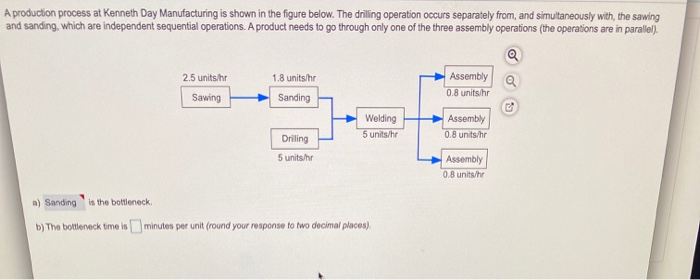A production process at Kenneth Day Manufacturing is shown in the figure below. The drilling operation occurs separately from, and simultaneously with the sawing and sanding, which are independent sequential operations. A product needs to go through only one of the three assembly operations (the operations are in parallel). Q 2.5 units.hr 1.8 units/hr Assembly a Sawing 0.8 units/hr Sanding Welding 5 units/hr Assembly 0.8 units/hr Driling 5 units.hr Assembly 0.8 units/hr a) Sanding is the bottleneck b) The bottleneck...

• ### The Pullman​ Mfg., Inc.​ three-station work cell illustrated in the figure below has two machines at...

The Pullman​ Mfg., Inc.​ three-station work cell illustrated in the figure below has two machines at station 1 in parallel.​ (The product needs to go through only one of the two machines before proceeding to station​ 2.) Station 1Machine AStation 1Machine BStation 2Station 320 min/unit20 min/unit12 min/unit3 min/unit The​ manager, Ms.​ Hartley, has asked you to evaluate the system if she adds a parallel machine at station 2. ​a) The throughput time of the new work cell is 35 minutes...

• ### NEED ANSWER D. The Pullman Mfg., Inc. three-station work cell illustrated in the figure below has...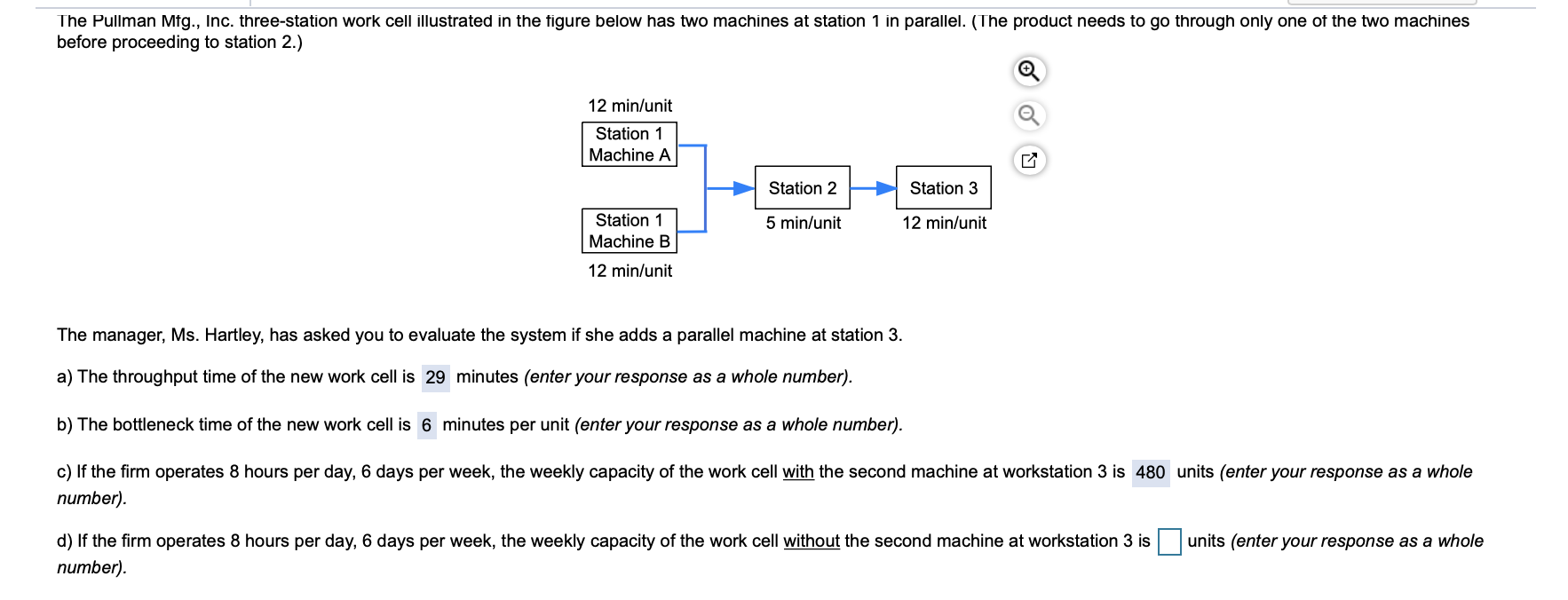NEED ANSWER D. The Pullman Mfg., Inc. three-station work cell illustrated in the figure below has two machines at station 1 in parallel. (The product needs to go through only one of the two machines before proceeding to station 2.) 12 min/unit Station 1 Machine A Station 2 Station 3 5 min/unit 12 min/unit Station 1 Machine B 12 min/unit The manager, Ms. Hartley, has asked you to evaluate the system if she adds a parallel machine at station 3....

• ### A production process at Kenneth Day Manufacturing is shown in the figure below. The drilling operation...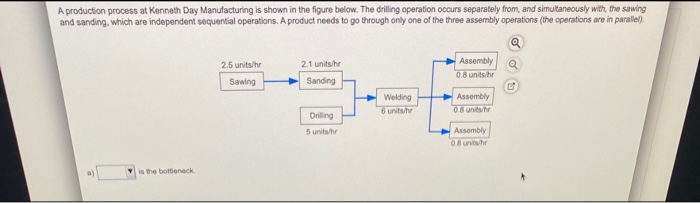A production process at Kenneth Day Manufacturing is shown in the figure below. The drilling operation occurs separately from, and simultaneously with the sawing and sanding, which are independent sequential operations. A product needs to go through only one of the three assembly operations (the operations are in parallel). Q 2.5 units/hr Sawing 2.1 units/he Sanding Assembly 0.8 unitsthe Welding 6 units.hr Assembly 0.8 units Drilling 5 unitshe Assembly 0.8 untui is the bottleneck 0.7 units/hr a) Drilling is the...

• ### . Assume that the following quantity discount schedule applies: Order Size Discount Unit Cost 0 to...

. Assume that the following quantity discount schedule applies: Order Size Discount Unit Cost 0 to 999 0% \$150 Over 999 \$5 per unit \$145    Annual demand is 5000 units, ordering cost is \$ 400 per order, and inventory holding cost is 10% of the cost of goods. What is the optimal quantity to order? What will be the Inventory Cost?

• ### The three-station work cell illustrated in the figure below has a product that must go through...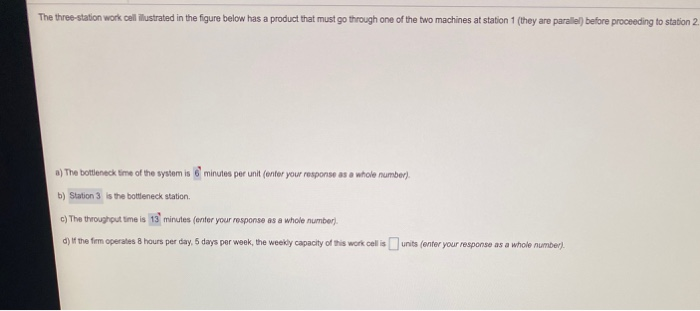The three-station work cell illustrated in the figure below has a product that must go through one of the two machines at station 1 (they are parallel) before proceeding to station 2. a) The bottleneck time of the system is 6 minutos per unit (onitor your response as a whole number). b) Station 3 is the bottleneck station c) The throughout time is 13 minutes (onter your response as a whole number). d) If the firm operates 8 hours per...

• ### Summer-Fun, Inc., produces a various commodities related to recreation and leisure. The production manager has developed...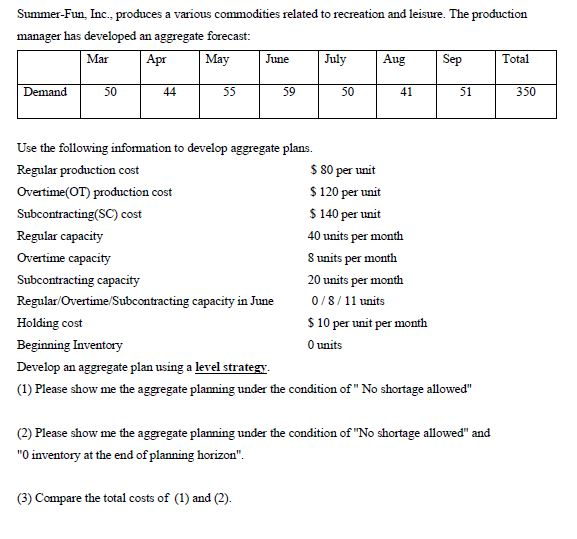Summer-Fun, Inc., produces a various commodities related to recreation and leisure. The production manager has developed an aggregate forecast: Apr May Aug Sep Total Mar June July Demand 50 1 44 | 55 | 59 | 50 | 41 51 350 Use the following information to develop aggregate plans. Regular production cost \$ 80 per unit Overtime(OT) production cost \$ 120 per unit Subcontracting(SC) cost \$ 140 per unit Regular capacity 40 units per month Overtime capacity 8 units per...

• ### The current aggregate demand requirements for a firm are shown below for the next six months:...

The current aggregate demand requirements for a firm are shown below for the next six months: Month May June July Aug Sept Oct Demand 270 250 250 250 280 300 The firm always plans to meet all demand. The firm currently has 270 workers capable of producing 270 units in a month (1 unit/worker). The workforce can be increased (at a cost of \$700 per worker) or decreased (at a cost of \$1,400 per worker). Inventory holding cost is \$175...

• ### Problem 13-4 The current aggregate demand requirements for a firm are shown below for the next...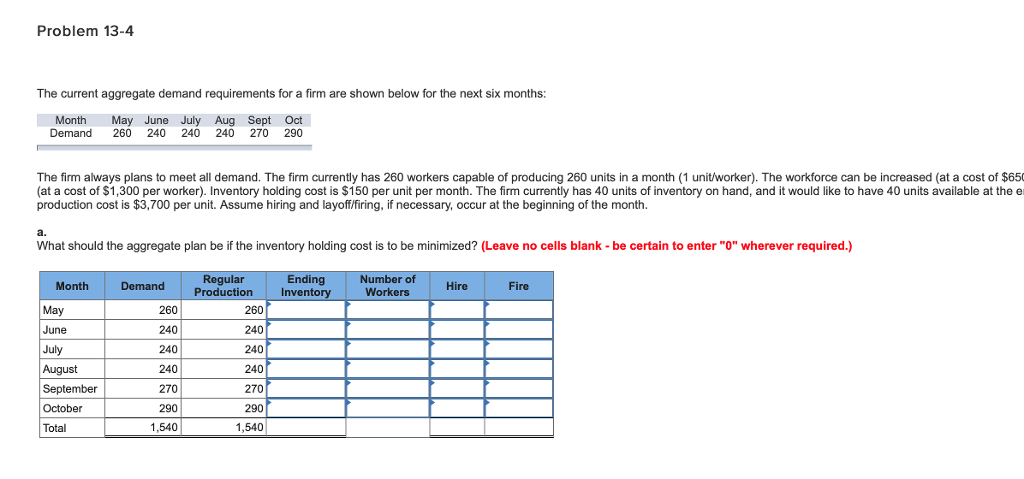Problem 13-4 The current aggregate demand requirements for a firm are shown below for the next six months MonthMay June July Aug Sept Oct Demand 260 240 240 240 270 290 The firm always plans to meet all demand. The firm currently has 260 workers capable of producing 260 units in a month (1 unit/worker). The workforce can be increased (at a cost of \$650 (at a cost of \$1,300 per worker). Inventory holding cost is \$150 per unit per...